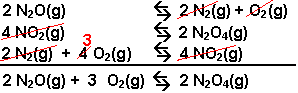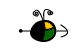Calculating K for a Reaction
Using Known K's for Other Reactions

K for a Reversed Reaction

The equilibrium expression written for a reaction written in the reverse direction is the reciprocal of the one for the forward reaction.

K' = 1/K

K' is the constant for the reverse reaction and K is that of the forward reaction.

Example:  What is the value of the equilibrium constant for the reaction 2 NO2(g)N2O4(g) at 100o C?

N2O4(g)2 NO2(g)   Kc = 0.212 @ 100oC?

The desired reaction is the reverse of the reaction for which the Kc is known.  The equilibrium expression is the reciprocal of that given.

K'c = 1/Kc = 1/0.212 = 4.72

Top

Reaction Coefficients Multiplied by a Number

If the coefficients in a balanced equation are multiplied by a factor, n, the equilibrium expression is raised to the nth power.

K' = (K)n

K' is the constant for the reaction multiplied by n and K is the constant of the original reaction.

Example:  What is the value of the equilibrium constant for the reaction, 2 I2(g) + 2 Cl2(g)4 ICl(g)?

½ I2(g) + ½ Cl2 (g)ICl(g)    Kc = 4.54 x 102 @ 25oC?

The desired reaction has been multiplied by 4.  The value of the equilibrium constant will be the 4th power of the given Kc.

K'c = Kc4 = (4.54 x 102)4 = 4.25 x 1010

Top

If two or more reactions are added to give another, the equilibrium constant for the reaction is the product of the equilibrium constants of the equations added.

K' = K1 x K2  . . .

K1 , K2, etc. represent the equilibrium constants for reactions being added together, and K' represents the equilibrium constant for the desired reaction.

Example: Calculate the value of Kc for the reaction:  2 NO(g) + Br2(g)2 NOBr (g) using the following information.

2 NO(g)N2(g) + O2(g)                            Kc1 = 1 x 1030
N2(g) + Br2(g) + O2(g)2 NOBr(g)          Kc2 = 2 x 10-27

The two equations can be added to yield the desired equation. The value of Kc for the reaction will be the product of the other two.

K'c = Kc1 x Kc2 = (1 x 1030)(1 x 10-27) = 2 x 103

Top

Calculations Incorporating Two or More of These Algebraic Manipulations

It is possible to combine more than one of these manipulations.

Example:   Calculate the value of Kc for the reaction:  2 N2O(g) + 3 O2(g)2 N2O4(g), using the following information.

 Equation Equilibrium Constant 2 N2 (g) + O2(g)2 N2O(g) Kc = 1.2 x 10-35 N2O4(g)2 NO2(g) Kc = 4.6 x 10-3 ½ N2(g) + O2(g)NO2(g) Kc = 4.1 x 10-9
These three equations can be combined to get the desired reaction.• Write the first equation backwards.  The K for this reaction will be the recipricol of the forward reaction.
 2 N2O(g)2 N2(g) + O2(g) Kc = 1/(1.2 x 10-35) = 8.3 x 1034
• Write the second equation backwards and multiply the coefficients by 2.  The K for this reaction will be the recipricol of the forward reaction squared.
 4 NO2(g)2 N2O4(g) Kc = 1/(4.6 x 10-3)2 = 4.7 x 104
• Use the third equation in the forward direction but multiplied by 4.  The K for this reaction will be the K of the given reaction raised to the fourth power.
 2 N2(g) + 4 O2(g)4 NO2(g) Kc = (4.1 x 10-9)4 = 2.8 x 10-34
• Check to see that the three equations yield the desired equation when added together.  The equilibrium constant for the desired equation will be the product of the constants for the three equations combined.

Kc = (8.3 x 1034)(4.7 x 104)(2.8 x 10-34) = 1.1 x 106

Top